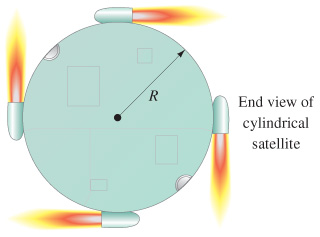# Problem: To get a flat, uniform cylindrical satellite spinning at the correct rate, engineers fire four tangential rockets as shown in the figure.If the satellite has a mass of 3600 kg , a radius of 4.6 m , and the rockets each add a mass of 230 kg , what is the required steady force of each rocket if the satellite is to reach 33 rpm in 5.3 min , starting from rest?

###### FREE Expert Solution

Torque:

$\overline{){\mathbf{\tau }}{\mathbf{=}}{\mathbf{r}}{\mathbf{F}}}$.

Now, the net torque due to the firing rocket is found as:

τ = RF + RF + RF + RF = 4RF

Torque can also be expressed as:

$\overline{){\mathbf{\tau }}{\mathbf{=}}{\mathbf{I}}{\mathbf{\alpha }}}$, where I is the moment of inertia and α is the angular acceleration.###### Problem Details

To get a flat, uniform cylindrical satellite spinning at the correct rate, engineers fire four tangential rockets as shown in the figure.

If the satellite has a mass of 3600 kg , a radius of 4.6 m , and the rockets each add a mass of 230 kg , what is the required steady force of each rocket if the satellite is to reach 33 rpm in 5.3 min , starting from rest?